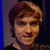+10

# Use "sticky headers" to display current class and method

Desmond Brand il y a 7 ans
This idea is not specific to Python, and would probably be useful if implemented generally based on indentation, but I'm going to describe it in terms of Python for clarity.

I often use "go to definition" to jump directly to a method. But it's sometimes hard get context because I can't see which class the method is inside of - I have to scroll up, sometimes really far, to see what the class is.

My idea is to reserve one line at the top of screen for each level of indentation of the current line. Then show the "parent" line as a "sticky header" at the top of the editor. The "sticky header" is often found in iOS, for example the contact list. Here's a video that illustrates the point. The class would always be visible on the first line, the method visible on the second. Maybe it could be useful to go further than this and make if statements and loops sticky, but I'm not sure about that.

Here's an example of the code review tool Phabricator doing this. Note the line number of the class is 185, but the first line shown is 201. The rest of the body has been collapsed so that the class line remains visible for context.

For a more extreme example, consider the following 5 line display. Obviously on such a small display the lines lost to headers reduce the amount of space you have to see the actual body, but a small example makes it easiest to see how this would work.
```class LineZero(object):
first_line = "bar"
def second_line(self):
third_line = "baz"
fourth_line = 42
```
Right now if you scroll that, the class line disappears from view:
```    first_line = "bar"
def second_line(self):
third_line = "baz"
fourth_line = 42
fifth_line = 5
```
I'm proposing that the class statement (as the root node in the tree of indentation) become "sticky", so instead the first line of the class scrolls out of view like this:
```class LineZero(object):
def second_line(self):
third_line = "baz"
fourth_line = 42
fifth_line = 5
```
Then if you scrolled one line further, the method line would be sticky, and the first line of the method body scrolls out of view:
```class LineZero(object):
def second_line(self):
fourth_line = 42
fifth_line = 5
sixth_line = None
```